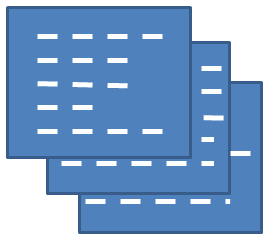In this help we continue with our example model that is tasked with generating code for faq pages. We will be going into greater detail on how to code the generate_code method that is required for every model. Before resuming our faq example we will discuss some general techniques that pertain to code generation. Recall that the main purpose of the generate_code method is to generate a string containing a program or other module to be saved to the file system. The model does not handle the actual saving, this is done by the GenHelm base classes.

### Common Generation Techniques

#### Line Concatenation

For code readability, we normally end each line of generated code with a newline character. Usually the constant PHP_EOL will serve this purpose. Occasionally, you need to use "\n" instead. Here we see a couple of patterns you can use.

In the first pattern, we initialize an empty string and then continue to concatenate onto it as in:

``````\$code = '';
\$code .= 'line 1'.PHP_EOL;
\$code .= 'line 2'.PHP_EOL;
\$code .= 'etc.'.PHP_EOL;``````

Another way to achieve the same thing would be to code something like:

``````\$line[] = 'line 1';
\$line[] = 'line 2';
\$line[] = 'etc.';
\$code = implode(PHP_EOL,\$line).PHP_EOL;``````

PHPs heredoc and nowdoc string assignment may also come in handy when generating certain strings. Keep in mind that heredoc strings may contain variables whose values will be substituted into the assigned string whereas nowdoc should be used in cases where you don't want any string parsing/substitution performed.

When you code your own models, use whatever string formulation techniques that work best in your situation.

#### Minimize Redundant Code

A common use case involves generating (or not generating) optional parameters. Let's say our code needs to generate one of the following depending on whether the user specified a value for d:

``````\$this->some_function(\$a,\$b,\$c);
\$this->some_function(\$a,\$b,\$c,\$d);``````

Avoid generating this with an if statement such as:

``````if (empty(\$this->dvalue)) {
\$code .= '\$this->some_function(\$a,\$b,\$c);'
}
else {
\$code .= '\$this->some_function(\$a,\$b,\$c,\$d);'
}``````

Using this technique you need to repeat the generation of the method call twice which makes the code harder to maintain. A better strategy would be to code it like this:

``````\$optional := empty(\$this->dvalue) ? '' : ',\$d';
\$code .= '\$this->some_function(\$a,\$b,\$c'.\$optional.');'``````

With this approach, the function parameters only need to be referenced once by the generator and the optional parameter is handled by a simple empty string concatenation.

#### Using PHP's var_export Function

Often generated code needs to render a value entered by the user on the specification form. var_export is a very powerful function that renders the PHP code to represent a variable value. Here is some sample code that illustrates the use of this function.

``````\$boolean = true;
\$string = 'Don\'t "mess" up';
\$integer = 123;
\$float = 456.78;
\$array = array('a','b','c');
\$keyed_array = array('fruit' => 'apple', 'vegetable' => 'broccoli');
return 'boolean: '.var_export(\$boolean,true).'<br />'.
'string: '.var_export(\$string,true).'<br />'.
'integer: '.var_export(\$integer,true).'<br />'.
'float: '.var_export(\$float,true).'<br />'.
'array: '.var_export(\$array,true).'<br />'.
'keyed_array: '.var_export(\$keyed_array,true);``````

And here we see the results of the returned string rendered in a browser:

`boolean: truestring: 'Don\'t "mess" up'integer: 123float: 456.78array: array ( 0 => 'a', 1 => 'b', 2 => 'c', )keyed_array: array ( 'fruit' => 'apple', 'vegetable' => 'broccoli', )`

Notice how the function has correctly escapes the quotes in our string while the boolean and numeric fields are unquoted as expected.

The var_export function generates an extra comma after the list of array values but this does not have any ill effect.

Be sure to remember to pass true as the second parameter to var_export, otherwise the result will be echoed, which we don't want.

### Using the basename function

When generating classes, often the name of the stowed specification coincides with the class name to be generated. The specification name is passed to the model using the variable \$spec_name. Keep in mind that the GenHelm user is allowed to enter a path when stowing a specification. For example, stow helper/country. If we use \$spec_name as the class name in such a case, the code will not compile. You will need to define a strategy for turning \$spec_name into a valid class name. One way might be to simply drop the path. An easy way to do this is to use the basename function as we see in this example:

``````\$code .= 'require_once CLASS_PATH.\'flexgrid_base.php\';'.PHP_EOL;
\$code .= 'class '.basename(\$spec_name).' extends \\flexgrid_base {'.PHP_EOL;``````

### Sharing Information Across Methods

The Scalar Fields and Flexgrid definitions are used to collect parameters that influence the code that gets generated by the model. All of these parameters must appear on the form presented to the user. Sometimes your models may need additional parameters used to share values between method calls. For example, the spec_validation method may need to lookup certain information as part of the validation processing. Later, during the generate method, this same information might be needed to generate portions of code. Rather than having to reconstitute this information, it is possible to define properties of the model that are used internally to share information across methods. Properties are defined within the Custom Code section using Section Type property and Location After Last Sibling as shown here: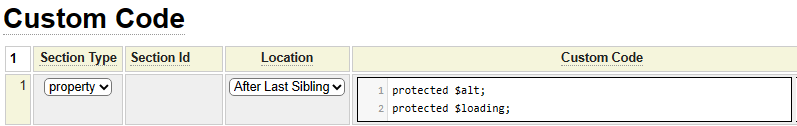Properties should be defined as the first section.

Here we show an example wherein the spec_validation method determines whether the spec contains an alt property and saves this within the \$this->alt property. This property is later used within the generate method.

``````function spec_validation(\$spec_name) {
// Alt is required for W3 validation
\$properties = \$this->get_spec_row('image_property_flexgrid');
\$this->alt = false;
if (\$properties !== false) {
foreach (\$properties as \$id => \$values) {
if (\$id === 'alt') {
\$this->alt = true;
break;
}
}
}
}
function generate() {
...
if (\$this->alt === false) {
\$properties['alt'] = array('value'=>\$this->spec_description);
\$save_alt = \$this->spec_description;
\$this->assign_message('image',2115,\$save_alt,'Specification description ":1:" will be used as alt.',
__LINE__.' '.__CLASS__);
}
...
}``````

## Modifying the Specification Programmatically

Sometimes the generate_code method may change the specification that was entered by the user. When this happens, the values of the specification in memory may deviate from the xml used to cache the specification. If you programmatially modify the specification you should always call the following base method to alert the GenHelm kernel to the fact that the specification has changed:

``\$this->set_spec_altered();``

## The generate_code Method

Let's get back to our model example that started on this page.

Recall that the purpose of the model is to generate code that will be included into the html_page_faq class. The generated code will look something like the .inc file shown here:

``````\$this->set_div_wrapper_properties('class="override"');
\$this->div_start();
true);
array('Answer to first question in section 2.','Another paragraph.'));
\$this->div_end();``````

Below we see the code for the generate_code function. Notice that we don't include the function name or opening and closing brackets since we are using the Section Id generate_code with Replace Body in the Location as shown here: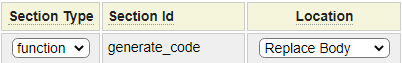``````\$generating = \$spec_name.'.inc';
\$code = \$this->get_object_banner(\$generating);
\$code .= \$this->gen_preamble();
\$prefix = '\$this->set_';
\$skip_div = false;
if (!empty(\$this->div_wrapper_properties)) {
if (\$this->div_wrapper_properties === '!') {
\$skip_div = true;
}
else {
\$code .= \$prefix.'div_wrapper_properties('.\$this->quote(\$this->div_wrapper_properties).');'.PHP_EOL;
}
}
}
if (!\$skip_div) {
\$code .= '\$this->div_start();'.PHP_EOL;
}
if (!\$skip_div) {
\$code .= '\$this->div_end();'.PHP_EOL;
}
\$code .= \$this->gen_postamble();
\$return[] = array('folder' => 'includes', 'subfolder' => 'page', 'name' => \$generating, 'contents' => \$code);
\$this->generate_common_page_info(\$return, \$spec_name);
return \$return;``````

Let's break down what this code is doing.

Recall that \$spec_name contains the name of the specification being stowed. We use this to derive the name of the .inc file we will be saving.

We use the local variable \$code to store the code we are generating.

### get_object_banner

We can call the inherited function get_object_banner to generate some comments at the beginning of the document. This will generate something like this:

`/* Program......: faqs.inc * Model........: faq * Specification: faqs.xml * Generated on.: 2021-12-06 * Generated by.: ssinclair * Description * Address common questions about our tarps.*/`

The description is taken from the spec description entered below the GenHelm command line as shown here: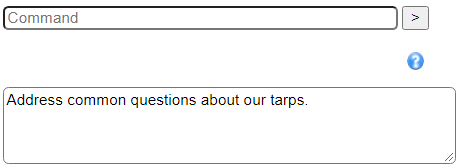### gen_preamble

Recall that every page-oriented model, like faq, provides the option of entering a preamble and postamble that will appear above and below the "main" page content. This is supported by these common properties: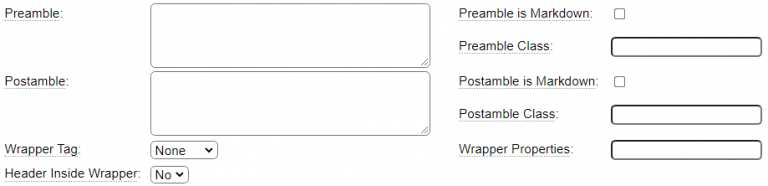You need to add some code to each page-oriented model to support these fields. This line inherits code that deals with the preamble rendering:

``\$code .= \$this->gen_preamble();``

### Model Specific Code

Since much of the code starts with \$this->set_ we put this into a variable named \$prefix.

#### Supporting the Outer div Tag

Normally the questions and answers are wrapped in a div tag. We allow developers to optionally specify the properties for this tag. We are also going to add a feature which will allow developers to not generate this div at all. This will be the behavior when the developer enters "!" as value for the div properties. So there could be one of three situations:

1. User enters ! in the div properties... Don't generate the div tag.
2. User enters something else in the div properties... generate a call to the set_div_wrapper_properties method passing the supplied properties.
3. User does not enter anything into the properties... generate the div tag but don't set any properties.

On this line we use the inherited function quote that will quote the supplied string and escape any quotes if the string, itself, contains quotes:

``\$code .= \$prefix.'div_wrapper_properties('.\$this->quote(\$this->div_wrapper_properties).');'.PHP_EOL;``

We could have also used PHPs var_export function nstead of the quote function for this purpose. We use the built-in variable PHP_EOL to concatenate the end of line character onto the code lines.

With this code we are going to generate the opening div (unless the user skipped this by entering ! in the div properties), then we call a function to generate the questions and answers (we will look at this later) then we generate the closing div.

``````if (!\$skip_div) {
\$code .= '\$this->div_start();'.PHP_EOL;
}
if (!\$skip_div) {
\$code .= '\$this->div_end();'.PHP_EOL;
}``````

### gen_postamble

As with the preamble, we call an inherited function to generate the postamble.

### Passing Back the Generation Directives

Let's review the final three lines in the generate_code function:

``````\$return[] = array('folder' => 'includes', 'subfolder' => 'page', 'name' => \$generating, 'contents' => \$code);
\$this->generate_common_page_info(\$return, \$spec_name);
return \$return;``````

Recall that the array that's returned to the caller contains one entry for every document to be generated. You may have noticed that all page-oriented models generate two documents as we see here: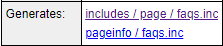We are going over how the first document is created but where does the second document come from?

This is handled by the call to the inherited method generate_common_page_info. Notice that we pass the \$return array to this method. This is passed by reference and the method generates the pageinfo document for us and adds this to the \$return array.

## Generating the Question and Answer Code

Next let's get into the details of the question_answer_code function. Much of this code involves interacting with the model_faq_flexgrid control. Here we see the definition of this control: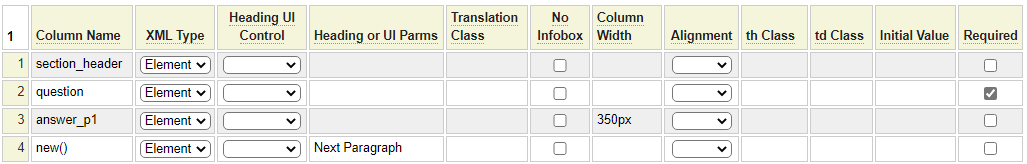One thing to note is that the control has 3 fixed columns named section_header, question and answer_p1. Additionally, the user can add any number of columns. These new columns will end up being numbered 4, 5, 6, etc. Also, flexgrids have a built-in column with a key of '#' which contains the row number.

Here we see the code that interacts with the flexgrid:

``````function question_answer_code() {
\$code = '';
\$auto_open = empty(\$this->auto_open) ? '' : ',true';
\$spec_row = \$this->get_spec_row('model_faq_flexgrid');
if (\$spec_row !== '') {
foreach (\$spec_row as \$cols) {
}
\$question = \$this->quote(\$cols['question']);
unset(\$cols['#']);
unset(\$cols['question']);
// everything left is an answer paragraph
foreach (\$cols as \$value) {
if (!empty(\$value)) {
}
}
}
}
else {
}
if (\$this->auto_open !== 'all') {
\$auto_open = '';
}
}
}
return \$code;
}``````

We will explain the key aspects of this code next.

To obtain the flexgrid as an array we use the inherited method get_spec_row passing the name of the flexgrid as in:

``\$spec_row = \$this->get_spec_row('model_faq_flexgrid');``

This will return an empty string if there are no rows in the grid, otherwise it will return a two-dimensional array such as the following:

``````array (
0 =>
array (
'question' => 'Question 1',
4 => 'Answer 1 Paragraph 2',
5 => 'Answer 1 Paragraph 3',
'#' => '1',
),
1 =>
array (
'question' => 'Question 2',
4 => '',
5 => '',
'#' => '2',
)
)``````

If there is a section header, we are going to generate a call to the add_header method, passing the name of the section header as shown here:

``````if (!empty(\$cols['section_header'])) {
}``````

We are using an inherited function named quote that similar to the var_export function for strings, that is, it will quote the string and escape any embedded quotes.

After extracting the section_header and and question from the array we unset these keys as well as the # key. After doing so, the only values that should remain in the array are the answer paragraphs. We cycle through these and create an array of answers here:

``````unset (\$answer);
// everything left is an answer paragraph
foreach (\$cols as \$value) {
if (!empty(\$value)) {
}
}``````

Next we generate the answer value as a string or an array and use this in the code that generates the call to the add_question method as shown here:

``````if (!isset(\$answer)) {
}
}
else {
}
if (\$this->auto_open !== 'all') {
\$auto_open = '';
}``````

In the earlier code the \$auto_open was set to either ',true' or an empty string. If all of the questions are to be auto opened we leave this value as ',true', otherwise we change it to an empty string so that only the first question is auto opened.

Finally, we return the generated code to the caller.

## Other Methods Models Can Implement

Although the generate_code method is the only required method that models must implement, there are other optional methods that models can implement to improve the user experience. Follow this link to learn about optional model methods.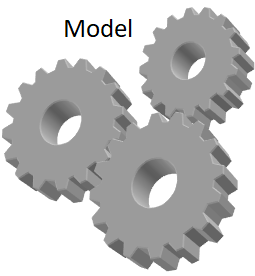🡇